﻿ Chapter 5D Abridged (Simpson's Rule)

## V.D Other Methods of Estimating The Definite Integral (Abridged)

 The development and study of numerical methods for estimating areas preceeds the development of the calculus. The notation and results of the calculus provides some support to a hopeful belief that perhaps the fundamental theorem of calculus ends the need for estimation of areas. Unfortunately it is not possible to find elementary function antiderivatives for many important functions like `{sin(x)}/x `and   `e^{-x^2}`. So the study of estimation techniques for definite integrals (sometimes described as the quadrature problem) continues today, even when current technology can achieve quite remarkable results. The search in numerical analysis today is for more efficient and effective algorithms that estimate a definite integral.
Preface: The evaluation form of the fundamental theorem of calculus allows us in theory to find the value of any definite integral of a continuous function f as long as there is a computable primitive function `F` where ` F '(x)=f(x)` . The description of a primitive function for the function f in the derivative form of the fundamental theorem is as an integral `F(x)=int_a^x f(t)dt` .
Unfortunately this description of a primitive function using the integral for f begs the question of whether this function is computable in some direct way. Certainly most of the examples we have discussed have had primitive functions that can be described using elementary functions, but there are many important functions for which there is no easily computable elementary primitive function. For example the function `e^{-x^2}`, which plays an important role in the study of probability, does not an elementary primitive function. In some situations the value of the integrand may not be described algebraically, and only graphical or numerical information about the function may be available to estimate the related definite integral. Many rational functions do not have an easily described primitive function because of the practical difficulties of finding the precise roots of the polynomials. (See Chapter VII for more on integrating rational functions.) In fact, sometimes a primitive function defined by the integral becomes so important to applications that it is named and studied as carefully as the elementary trignometric functions. One very important example of this type in probability theory is the distribution function called the error function, denoted erf, defined by
erf`(x)=2/{sqrt(pi)}int_0^x e^{-t^2}dt`.

 The part of mathematics that is concerned with developing and analyzing algorithms for computing both exact and approximate values of numbers like the definite integral is called numerical analysis.
Since the definite integral was defined initially using estimates and limits, it is sensible to think that there might be other methods giving more accurate estimates of the definite integral for less effort. In this section we will investigate some alternatives for estimating the definite integral. In the process we will try to understand both the motivation for these estimates and the justification for believing these estimates actually do approximate the definite integral. We will look at some examples where we can find the definite integral's value exactly using the fundamental theorem of calculus. These examples provide some foundation for comparing the exact result with estimates to see how well a particular method performs when the result is known and comparing the performance of different algorithms. In other examples the fundamental theorem of calculus will not help evaluate the definite integral. In these cases it is truly necesssary to find more efficient methods for approximating its value and knowing something about the accuracy of these estimates.

• General Principles: The key motivations for estimating the definite integral are geometric or physical, connected to the interpretation of the definite integral for positive functions visually as an area or dynamically as the estimation of the distance travelled by an object moving. In either case the ideas first use a model for the estimation on a single interval. This model is then reproduced to estimate the integral for sub-intervals of shorter length. These estimates are accumulated to a sum of estimates which provides the improved estimate of the integral for the original interval. In some sense the estimates work by combining two slogans: "what's good for one is good for all" and "the more the merrier!" The accumulation of many small estimates each determined in the same fashion with small errors generally improves the quality of the approximation. So the fundamental approach to estimations of the definite integral follows a 3 step procedure: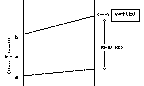• Single Interval Estimate Method: Choose a method to make an estimate of the area (or distance travelled) for the single interval [a,b] based on some limited but computable information about the function f for that interval.
• For example, use the area of the rectangle with height `f(b)` and base `b-a`, so we would estimate with `f(b) (b-a)`. See Figure 1. This method uses the height of the graph of the function at the right hand endpoint, `f(b)`, for the estimation of the area of the rectangle. This is in contrast to the Euler sum which uses the height at the left hand endpoint of the interval, `f(a)`.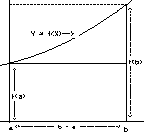•
• Or equivalently, use the distance travelled at velocity `f(b)` for time `b-a` to motivate the same estimate, `f(b) (b-a)`. See Figure ***. In the motion interpretation this uses the velocity at the arrival time, `f(b)`, to estimate the distance travelled during the time interval instead of the velocity at the initial time, `f(a)`, as used by Euler's method.
• Partition: Cut (partition) the given interval `[a,b]` into N pieces of the same size,`{b-a}/N=dx=Delta x`, and use the estimation method to estimate the area (distance travelled) for these smaller intervals and accumulate these estimates by adding to arrive at an estimate for the total area (or distance travelled). This sum may be simplified for computation using some algebra.
• Continuing the example, we would use intervals `[x_k, x_{k+1}]` where `x_k=a+k Delta x` for `k`= 0, 1, 2,..., N. Evaluate f at the right hand endpoint, `x_k`, when `k` = 1, 2, 3,... , N and multiply by the length `Delta x`, and then add these to give the estimate
• `f(x_1)Delta x +f(x_2)Delta x +...+ f(x_N)Delta x  =Sigma_{k=1}^N f(x_k)Delta x`
This formula for the estimate can be simplified by recognizing the common factor of  `Delta x`. So the estimate can be computed with the formula
`Delta x [f(x_1)+f(x_2) +...+ f(x_N)] = Delta x Sigma_{k=1}^N f(x_k)`

The graphical visualization is very suggestive here. A positive continuous function gives a picture with a region in the plane cut into N pieces, each estimated by a rectangle with base of length `Delta x` and height determined by the graph of the function at the right hand endpoint. See Figure ***. This estimate is called the right hand endpoint (Euler) estimate.

• Justify: We would like to justify that these estimates actually approach the desired integral as N gets large. To do this we connect our method either to the defining approximations of the definite integral or to some other estimate known to approximate the definite integral.
• In the example, the estimating sum `Sigma_{k=1}^N f(x_k)Delta x`  can be compared with the euler sum
`Sigma_{k=0}^{N-1}f(x_k) Delta x`
used to define the definite integral. The difference between these two estimates for the same number of segments, N, is
`Sigma_{k=1}^N f(x_k)Delta x` - `Sigma_{k=0}^{N-1}f(x_k) Delta x = f(x_N)Delta x - f(x_0)Delta x = (f(b)-f(a))Delta x.`
.
• Again the area interpretation can be helpful here. See Figure***.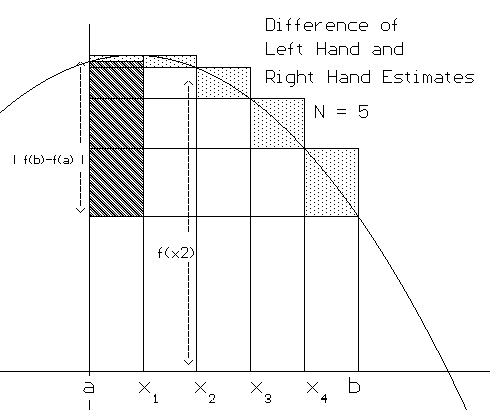Now when `N` is large, `Delta x =  {b-a}/N ->0` , so the difference between these two estimates is also close to 0. The definition of the definite integral tells us that when the definite integral exists, `Sigma_{k=0}^{N-1}f(x_k) Delta x -> int_a^b f(x) dx`.
• Hence the estimate `Sigma_{k=1}^{N}f(x_k) Delta x -> int_a^b f(x) dx.`
Simpson's Rule [Weighted average- quadratic interpolation]
Simpson's Rule for estimating `int_a^b f(x) dx` is developed by using quadratic functions (parabolas) to estimate the integrand f. There are many quadratic functions `P(x)=Ax^2+Bx+C` where `P(a)=f(a)` and `P(b)=f(b)` and whose graph therefore coincides at two points with the graph of `f`. To determine a single quadratic function for Simpson's rule, we consider the unique quadratic that also agrees with `f` at the midpoint `m=(a+b)/2`, that is, `P(m) = f(m)`.
Thus for the initial single interval estimate we approximate `int_a^b f(x) dx` with `int_a^b P(x) dx  = int_a^b Ax^2 + Bx+ C dx` where A, B, and C are determined by `P(a)=f(a), P(b)=f(b)`, and `P(m)=f(m)`.[ See Figure V.D.1.]
Now  `int_a^b Ax^2 + Bx+ C dx = {Ax^3}/3 + {Bx^2}/2 + C| _a^b`
` {A(b^3-a^3)}/3 +{B(b^2-a^2)}/2 +C (b-a) = {(b-a)}/6 [2A(b^2+ba+a^2) +3B(b+a) + 6C].`

When we plug `a, m,` and `b` into `f(x)` we obtain
`f(a) = Aa^2 + Ba + C`,
`f(m) = A ({a+b}/2)^2 + B{a+b}/2 + C = 1/4[Aa^2 + 2Aab + A b^2 + 2B( a+b) + 4C]`,
`f(b) = Ab^2 + Bb + C`.

With substitution and a little algebraic manipulation we arrive at the following rather simple equation relating the estimating quadratic integral to the values of `f` at `a`,` m`, and `b`.
`int_a^b Ax^2 + Bx + C dx = {b-a}/6 [f(a) + 4f(m) + f(b)].`

If `h={b-a}/2` and `a=x_0, m=x_1,` and `b=x_2` then this becomes

`int_a^b Ax^2 + Bx + C dx = h/3 [f(x_0) + 4f(x_1) + f(x_2)].`

To generalize this work we partition the interval `[a,b]` into `2k=N` subintervals of length `h=(b-a)/{2k}=(b-a)/N` by letting `x_i=a+ih`. We estimate the integral of f for each subinterval as we just did so that for each of the `k` intervals `[x_ {2i},x_{2i+2}]` we have

`int_{x_{2i}}^{x_{2i+2}}f(x)dx~~int_{x_{2i}}^{x_{2i+2}} Ax^2 + Bx + C dx = h/3 [f(x_{2i}) + 4f(x_{2i+1}) + f(x_{2i+2})].`
or
`int_{x_{2i}}^{x_{2i+2}}f(x)dx~~int_{x_{2i}}^{x_{2i+2}} Ax^2 + Bx + C dx = {b-a}/{6k} [f(x_{2i}) + 4f(x_{2i+1}) + f(x_{2i+2})].`

Adding these k estimates together gives us Simpson's Rule:
`int_a^b f(x)dx =Sigma_{i=0}^{k-1} int_{x_{2i}}^{x_{2i+2}}f(x)dx~~Sigma_{i=0}^{k-1}{b-a}/{6k} [f(x_{2i}) + 4f(x_{2i+1}) + f(x_{2i+2})].`
or
`int_a^b f(x)dx ~~{b-a}/{6k}Sigma_{i=0}^{k-1} [f(x_{2i}) + 4f(x_{2i+1}) + f(x_{2i+2})].`

This formula can be expressed in many different ways illustrating how to organize the required calculations efficiently. Here are a few alternatives:
`int_a^b f(x)dx ~~{b-a}/{6k} [f(x_{0}) + 4f(x_{1}) + 2f(x_{2}) + 4f(x_3)+ ...+ 2f(x_{2k-2}) + 4f(x_{2k-1}) + f(x_{2k})].`

Recalling that `N = 2k` gives another form usually denoted  `S_N`:
`int_a^b f(x)dx ~~{b-a}/{3N} [f(x_{0}) + 4f(x_{1}) + 2f(x_{2}) + 4f(x_3)+ ...+ 2f(x_{N-2}) + 4f(x_{N-1}) + f(x_{N})].`

Example V.D.1:Use Simpson's Rule with N = 4 to estimate`int_0^2 x^3 dx`.

Solution: Begin as usual by finding `{b-a}/N = {2-0}/4=1/2`. Thus `x_0= 0, x_1= 0.5, x_2= 1, x_3 = 1.5`, and `x_4 = 2`. Following the last formula for Simpson's Rule we have

`int_0^2 x^3dx ~~{2-0}/{3*4} [0^3 + 4(0.5^3) + 2(1^3) + 4(1.5^3)+ 2^3]`

`= 1/6 [0.5 + 2 + 13.5 + 8] = 1/6 24 = 4.`

Note that in this case , `int_0^2 x^3dx = 1/4 x^4| _0^2 = 16/4 = 4` so Simpson's Rule was better than just an estimate!

It is sometimes useful to organize the computations for an application of Simpson's Rule in a table. Here is a table showing the computations of the last example.
 xi `f(x)=x^3 Simpson Factor Summand 0 0 1 0 0.5 0.125 4 0.5 1 1 2 2 1.5 3.375 4 13.5 2 8 1 8 Simpson Sum = 24 Simpson Estimate = `1/6` Sum = 4

You can also look at a spreadsheet for `f (x) = x^4` .

Relating The Trapezoidal, Midpoint, and Simpson's estimates.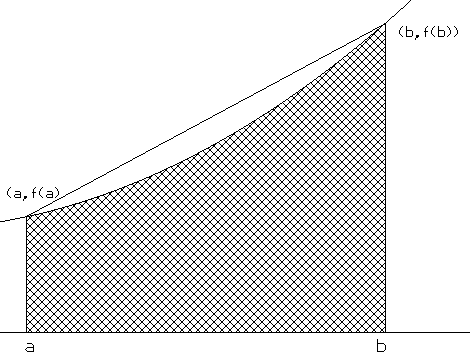Consider Figure 5 in which the graph of an increasing concave up function bounds a region R along with the X axis and the lines `X=a` and `X=b`. Because the graph is concave up, the line connecting the points `(a,f(a))` and `(b,f(b))` lies above the curve and the trapezoid formed using that line contains the region R.
Thus  `int_a^b f(x)dx <{b-a}/2 [f(a)+f(b)] =T`
Letting `m = (a+b)/2` in Figure 6 we consider another trapezoid, formed by the line tangent to the graph of `f` at the point `(m,f(m))`. Again using the concavity of `f` we can see this line lies below the curve so that the trapezoid is contained within the region R. Since `m` is the midpoint of the interval, the symmetry of the geometry for the trapezoid leads immediately to its area being `(b-a)f(m) = M`.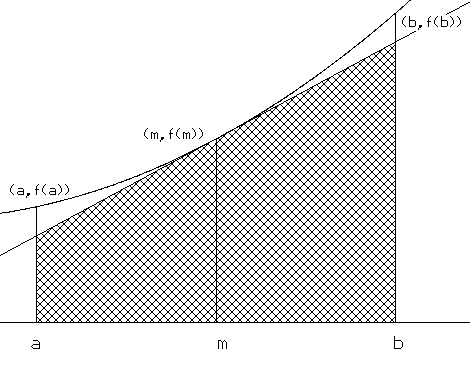Thus `M= (b-a)f(m) < int_a^b f(x)dx <{b-a}/2 [f(a)+f(b)] =T` . It appears that when a function is concave up, the definite integral lies between the midpoint and trapezoid estimates. Similar analysis works for a function that is concave down on an interval, leading to
`M= (b-a)f(m) > int_a^b f(x)dx >{b-a}/2 [f(a)+f(b)] =T`
. Again the definite integral lies between the midpoint and trapezoid estimates. So as long as we have a function that can be analyzed in sections where the concavity is well determined to be the same we have that the definite integral will be between the trapezoidal and midpoint estimates.

When the concavity is not the same throughout the interval there is still some relation between these three estimates. If we consider Simpson's single interval estimate for the interval [a,b] using only algebra we have that
`{b-a}/6 [f(a) + 4f(m) + f(b)] =1/3(b-a){f(a)+f(b)}/2 + 2/3 (b-a)f(m) = 1/3T +2/3M`

This result, being from algebra, does not depend on the figure or the convexity of the function.

From the way we construct our more general estimates (by partitioning the interval `[a,b]` and accummulating the single subinterval estimates) we can demonstrate the same algebraic relation of Simpson's Rule to the trapezoidal and midpoint estimates, namely
`S_N=(1/3) T_k + (2/3) M_k ` where `N=2k`.(*)
Using this algebraic relation we can see that if `T_k < M_k` then
`T_k = (1/3) T_k + (2/3) T_k < (1/3)T_k + (2/3) M_k = S_N`
and we also have that
`S_N =(1/3) T_k + (2/3) M_k < (1/3) M_k + (2/3) M_k = M_k. `

Likewise when `T_k > M_k` then

`T_k = (1/3) T_k + (2/3) T_k > (1/3)T_k + (2/3) M_k = S_N`
and
`S_N =(1/3) T_k + (2/3) M_k > (1/3) M_k + (2/3) M_k = M_k`.

[You might want to  look at a spreadsheet  comparing these methods of calculations for `f (x) = x^4` .]

So from the algebra we have that `S_N` is between `T_k` and `M_k` without reference to the concavity of `f`.Furthermore using (*) we can see easily that as `N->oo`,
`S_N ->1/3 int_a^b f(x) dx + 2/3int_a^b f(x) dx =int_a^b f(x) dx.` .